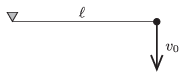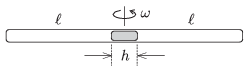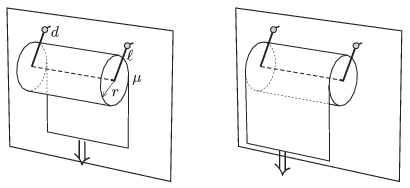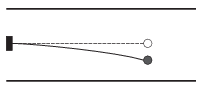Mathematical and Physical Journal
for High Schools
Issued by the MATFUND Foundation
 Already signed up? New to KöMaL?

# KöMaL Problems in Physics, May 2014

Show/hide problems of signs:## Problems with sign 'M'

Deadline expired on June 10, 2014.

M. 342. Measure the instantaneous speed of a table-tennis ball, which was dropped in static air, and plot the speed both as a function of the distance covered and as a function of the elapsed time. What will the terminal speed of the ball be?

(6 pont)

statistics## Problems with sign 'P'

Deadline expired on June 10, 2014.

P. 4638. An object is projected up at an initial upward speed of 12 m/s on the Moon. What will the speed of the object be at the moment when the half of the time of the upward motion elapsed? And what will its speed be when it is halfway of its upward motion?

(3 pont)

solution (in Hungarian), statistics

P. 4639. A uniform density cube of mass $\displaystyle m=2.7$ kg is on the horizontal tabletop. What should the least force be, in order that the cube turns about one of its edge? The coefficient of static friction is big enough, so the cube does not slide while it is turning. What is the least value of the coefficient of static friction?

(4 pont)

solution (in Hungarian), statistics

P. 4640. The internal energy of an ideal gas is the sum of the kinetic energies of the randomly moving molecules of the gas. The change in the internal energy of the gas is indicated by the change of the temperature of the gas. When the gas is given some energy in an ordered way, then it is called work, and if the energy is given in a disordered way then it is called thermal energy transfer. Which characteristics (states) of the ideal gas, the mass of which is kept constant, remain the same

$\displaystyle a)$ if only thermal energy is added;

$\displaystyle b)$ if only work is done;

$\displaystyle c)$ if the work done by the gas is continuously the same as the thermal energy added to the gas?

(4 pont)

solution (in Hungarian), statistics

P. 4641. An inclined plane of angle of elevation $\displaystyle \alpha$ is placed to a horizontal surface, and then an object of mass $\displaystyle m$ is placed to the top of the inclined plane. The small mass can slide on the slope without friction. With the help of the horizontal plane it is assured that the slope accelerates in the vertical direction at an acceleration of $\displaystyle a<g$.

$\displaystyle a)$ Determine the horizontal and the vertical components of the acceleration of the object sliding down the slope, and the equation of the path of the sliding object. (The object is always in contact with the slope.)

$\displaystyle b)$ How long does it take for the object to reach the bottom of the slope, if the length of the slope is $\displaystyle L$?

$\displaystyle c)$ What is the force exerted by the object on the slope?

Data: $\displaystyle \alpha=30^\circ$, $\displaystyle L=1.5$ m, $\displaystyle m=4$ kg, $\displaystyle a=6~\rm m/s^2$.

(4 pont)

solution (in Hungarian), statistics

P. 4642. A simple pendulum of length $\displaystyle \ell=20$ cm, is deflected to the horizontal, and is given an initial vertically downward speed of $\displaystyle v_0=2$ m/s. What is the angle between the thread and the vertical at the moment when the thread becomes tight again right after that it was loose?(5 pont)

solution (in Hungarian), statistics

P. 4643. Both ends of a horizontal thin tube are sealed. In the middle of the tube there is a Mercury thread of length $\displaystyle h$. The length of the air columns in both ends of the tube is $\displaystyle \ell$, and the pressure of the air in both parts is the gauge pressure of a Mercury column of height $\displaystyle H$. The tube is put into a centrifuge, which has a vertical axle, and is rotated at an angular speed of $\displaystyle \omega$.

What can the period of the oscillatory motion of the Mercury column be, provided that the temperature is constant and the oscillation is small? It can also be assumed that $\displaystyle h$ is small with respect to $\displaystyle \ell$, so the Mercury column does not break.(5 pont)

solution (in Hungarian), statistics

P. 4644. A roll of paper of radius $\displaystyle r=55$ mm is fixed to the wall of the bathroom. The roll is held by a frame of wire with two hinges, such that the hinges are at the same height and are at a distance of $\displaystyle d=15$ mm from the wall. The two side edges of the frame both has the length of $\displaystyle \ell=90$ mm, and this is the distance between the hinges and the axis of the paper roll. The paper roll can be rotated about the middle part of the frame with negligible friction. Due to its weight the structure touches the wall when it is at rest. The coefficient of friction between the tiles of the wall and the paper is $\displaystyle \mu=0.2$. The end of the paper is pulled at a uniform downward speed.

$\displaystyle a)$ In which case is it necessary to exert a greater force, if the free end of the paper is next to the wall, or if it is further away from the wall?

$\displaystyle b)$ What is the ratio of the two forces?

$\displaystyle c)$ What is this ratio if the radius of the paper roll is $\displaystyle r=100$ mm?(5 pont)

solution (in Hungarian), statistics

P. 4645. An object of mass 0.1 g is fixed to the end of a thin, initially horizontal quartz thread. The declination of the thread is 0.2 mm. The structure is placed into the space between the horizontal plates of a capacitor as shown in the figure. The distance between the plates is 3 cm. The object is given a charge of $\displaystyle 10^{-7}$ C.

$\displaystyle a)$ What should the applied voltage across the plates of the capacitor be in order that the quartz thread gains back its initial undeformed equilibrium position?

$\displaystyle b)$ The motion of the object is started with turning on the previously calculated voltage across the plates. What is the amplitude and the frequency of the initiated oscillatory motion?(4 pont)

solution (in Hungarian), statistics

P. 4646. What is the shape of the rounded end of that glass rod, which converges all the light rays entering onto the round glass surface parallel to the axis of the rod to one point inside the rod? Determine the equation of the surface as a function of the refractive index of the glass $\displaystyle n$ and the focal length $\displaystyle f$.

(5 pont)

solution (in Hungarian), statistics

P. 4647. An ,,observer'' moving at a speed of $\displaystyle \boldsymbol v_0$ in uniform magnetic field of magnetic induction of $\displaystyle {\boldsymbol B}_0$, detects an electric field of $\displaystyle {\boldsymbol E}_0= {\boldsymbol v}_0\times {\boldsymbol B}_0$, if $\displaystyle v_0\ll c$.

Does the opposite phenomenon exist? Does the observer moving in uniform electric field detect magnetic field? (Only high-school knowledge is to be used.)

(5 pont)

solution (in Hungarian), statistics

P. 4648. Three thin insulating rods, which all can be considered infinitely long and which are in the same plane, crossing each other such that they form an oblige triangle, are charged uniformly to the same density of charge. Where should a point-like charge be put, if it is to be in equilibrium?

(6 pont)

solution (in Hungarian), statistics